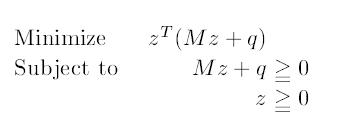# Linear Complementarity Problem

Linear Complementarity Problem (LCP) is a generalization of Linear Programming and a special case of quadratic programming. I stumbled upon LCP theory due to my interest in complexity problems in game theory and PPAD-completeness. As we will see these concepts are very closely related.

Let M be a$n \times n$ square matrix and q an n dimensional vector. Let LCP(q,M) be the following problem : Find two vectors w and z satisfyingLCP(q,M) consists of linear constraints and complementary conditions. Since$w{\geq}0, z{\geq}0$ the complementary conditions${w_i}{z_i}=0$ is equivalent to${w^T}{z}=0$. There is an obvious exponential time algorithm to solve LCP. For every i, set either$w_i=0$ or$z_i=0$ and solve the resulting system of linear equations. If one of these linear systems has a solution then the corresponding LCP is solvable. Deciding if a given LCP has a solution is NP-complete. The following exercise shows that LCP is a generalization of LP.

Exercise : Every LP can solved by solving a corresponding LCP, representing the complementary slackness of the LP.

LCP can also be expressed in the following equivalent form :Lemke’s algorithm is a “path-following” algorithm (similar to simplex algorithm) to solve LCP. Unfortunately, Lemke’s algorithm can sometimes fail to produce a solution even if one exists !! There are many special instances of LCP on which Lemke’s algorithm always produces a solution or a certificate that no solution exists.

As mentioned earlier, solving an LCP is NP-complete. What about special cases ? i.e., when the input matrix M is special.

• If M is a Positive Semi-Definite matrix, then LCP(q,M) can be solved in polynomial time. In fact, every LCP with a PSD matrix is a convex quadratic program and every convex quadratic program can be expressed as an LCP with a PSD matrix.
• If M is a Z-matrix, Chandrasekaran’s algorithm solves LCP(q,M) in polynomial time [Chandrasekaran’70].
• If M is a triangular P-matrix, LCP(q,M) can be solved in polynomial time by using a back substitution method.
• If M is a P-matrix, LCP(q,M) has a unique solution for every q.

Following is one of the coolest applications of LCP.

Exercise : Finding a Nash Equilibrium in a bimatrix game can be expressed as an LCP.

Lemke-Howson’s algorithm [Lemke,Howson’64] to solve a bimatrix game is known to take exponential number of steps in the worst case [Savani, vonStengel’04]. It is also known that finding Nash equilibrium in a bimatrix game is PPAD-complete [Chen,Deng’09].

Open Problems :

• The complexity of solving LCP with a P-matrix (P-LCP) is open for more than two decades !! P-LCP is known to be in PPAD [Papadimitriou’94]. Note that recognizing Z-matrices and PSD-matrices can be done in polynomial-time but recognizing P-matrices is coNP-complete [Coxson’94].
• Are there other interesting classes of matrices M for which LCP(q,M) is solvable in polynomial time ?
• Savani and von Stengel’s instance of bimatrix game has “full support mixed equilibrium”, which can easily solved using linear programming techniques. It is an open problem to construct an instance of a bimatrix game that does not have full-support mixed equilibrium and the Lemke-Howson algorithm takes exponential number of steps on this instance.
Savani and von Stengel’s instance of bimatrix game has full support.
It is open problem to construct an instance of bimatrix game that
does not have full-support mixed equilibrium and the Lemke-Howson algorithm
takes exponential number of steps.

References :

• [Chandrasekaran’70] R. Chandrasekaran. “A Special Case of the Complementary Pivot Problem“, Opsearch, 7(1970) 263 268.
• [Coxson’94] G. E. Coxson. The P-matrix problem is co-NP-complete. Math. Programming, 64(2):173–178, 1994.
• [Chen,Deng’09] Xi Chen, Xiaotie Deng, Shang-Hua Teng: Settling the complexity of computing two-player Nash equilibria. J. ACM 56(3): (2009)
• [Savani, vonStengel’04] Rahul Savani, Bernhard von Stengel: Exponentially Many Steps for Finding a Nash Equilibrium in a Bimatrix Game. FOCS 2004: 258-267
• [Lemke,Howson’64] Lemke, C. E. and J. T. Howson, Jr. (1964), Equilibrium points of bimatrix games. Journal of the Society for Industrial and Applied Mathematics 12, 413–423.
• [Papadimitriou’94] Christos H. Papadimitriou: On the Complexity of the Parity Argument and Other Inefficient Proofs of Existence. J. Comput. Syst. Sci. 48(3): 498-532 (1994)

## 3 thoughts on “Linear Complementarity Problem”

1.Rahul |

Nice post.

A solution to the last open problem, which is an extension of construction in the FOCS paper to non-square games, can be found in the journal version:

R. Savani and B. von Stengel (2006), Hard-to-Solve Bimatrix Games. Econometrica 74, 397-429.

To replace it, I have another one (related to your second open problem):

Parity games, mean-payoff games, and simple stochastic games, which are zero-sum games on graphs, all give rise to P-matrix (generalized) LCPs . The complexity of solving these games (for which the natural decision problems lie in NP and co-NP) is a long-standing open question.

Are these game easier than P-matrix LCPs? Certainly the “subexponential” LP-type methods of Matousek Sharir and Welzl and Kalai work for the games but not for P-matrix LCPs (due to possible cycles in the corresponding hypercube orientiations). For a starting reference, see

S. G. Vorobyov (2008), Cyclic games and linear programming. Discrete Applied Mathematics, 156 (11) pp 2195-2231.

Incidentally, P-matrix LCP lies in both PPAD and PLS, so is unlikely to be hard for either class.

•kintali |

Thanks Rahul. Your insights and the new open problem are quite interesting.

2.ILEGBEJIE, ANTHONY AGUELE |

I am just finding my way into the linear complementary problems. After some research in this area, I will start contributing. Thanks